# Load Profiles and their use in Electricity Settlement

v 4.0

 Load Profiles and Their use in Electricity Settlement Guidance Note

# Introduction

This document provides a broad overview of ‘Load Profiling’. It explains what a Load Profile is, how Load Profiles are created by the Profile Administrator (PrA), and how they are applied to the Balancing and Settlement Code (BSC). The use of ‘Load Profiles’ for electricity settlement was a product of the Electricity Pool ‘1998 Programme’ put in place to open up the electricity supply market to competition.

In order to avoid fitting Half-Hourly meters into all properties , it was decided that customers below 100 kW Maximum Demand would be settled using load profiles and readings from customers’ existing (non Half-Hourly) electricity meters. Eight basic types of profiles (Profile Classes) were created, each of which could be manipulated to model all the different metering configurations that exist.

Following the implementation of Modification P272 ‘Mandatory Half Hourly Settlement for Profile Classes 5-8’ in April 2017, the number of Profile Classes that require profiling reduced to four (Profile Classes 1-4).

# SECTION A

## What is a Load Profile?

Load Profile is a broad term that can refer to a number of different forms of data. It can refer to demand data, and consumption data, or to derived data types such as Regression and Profile Coefficients. However, all these data types have one thing in common; they represent the pattern of electricity usage for a customer segment of the electricity supply market.

A load profile gives the Half-Hourly (Settlement Period) pattern or ‘shape’ of usage across a day (Settlement Day), and year (Settlement Year), for the average customer of each Profile Class. It is the proportion of demand in each Settlement Period that matters in the Settlement system.

Figure 1 show an example of a typical daily demand pattern, and Figure 2 an example of a typical yearly demand pattern, for a domestic unrestricted customer (Profile Class 1):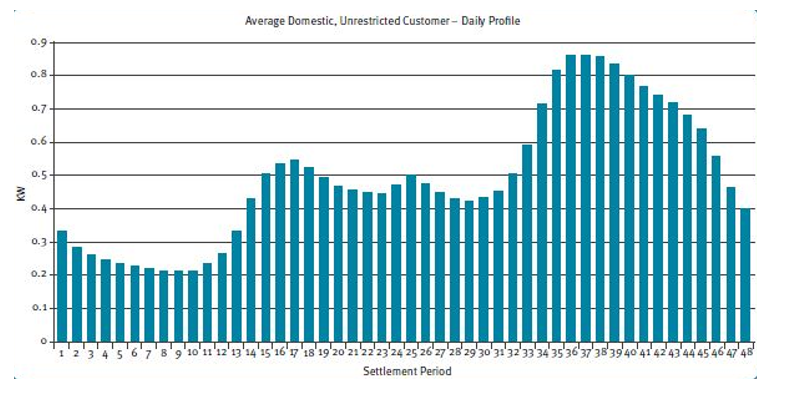Figure 1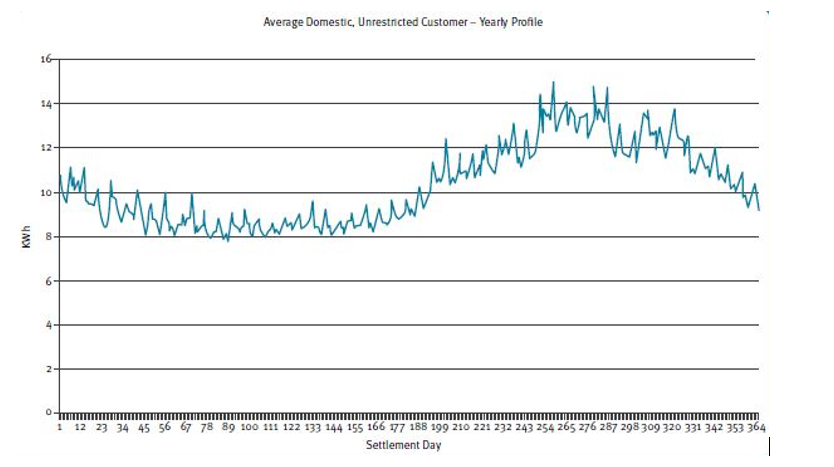Figure 2

Figure 1 shows the daily pattern of demand in kW, and Figure 2 shows the yearly pattern in kWh per day. The data provided to Settlement by the PrA is given in the form of Regression and Profile Coefficients, which are used to give the usage patterns for each category. The format and usage of the coefficients are discussed later in this document.

## The Profile Classes

The four generic Profile Classes represent large populations of similar customers, and are as follows:

• Profile Class 1 Domestic Unrestricted Customers (Single Rate)

• Profile Class 2 Domestic Economy 7 Customers (Two Rate)

• Profile Class 3 Non-Domestic Unrestricted Customers (Single Rate)

• Profile Class 4 Non-Domestic Economy 7 Customers (Two Rate)

# SECTION B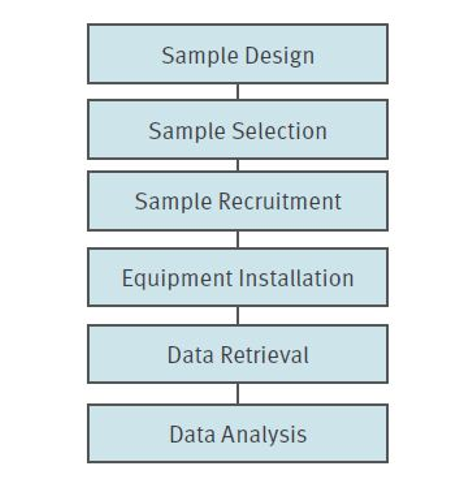Load Profile data is created by recording and analysing Half-Hourly demand data from a representative sample of non Half-Hourly customers, for each of the four Profile Classes. This section discusses the sample selection and data collection processes involved in creating a load profile. Figure 3 is a simplified model of the process, followed by a brief description of each stage.

Figure 3

## Sample Design

In order to design a sample, the required sample size must be agreed. There are two main drivers of this decision which need to be “traded off” against each other : Cost (the larger the sample size, the greater the expense at each stage) vs.Accuracy(the larger the sample size, the greater the accuracy)

A sampling variable is therefore required, and since we are modelling the average load shape the sampling variable is annual consumption (kWh).

Using a sampling fraction of 1 in n (e.g. 1 in 2000 for domestic customers) it is possible to draw a random primary sample from Supplier billing systems for each of the Profile Classes.

Using this information, a stratified sample can be defined - consumption bands. By setting the strata so customers within each band have less variance, greater accuracy can be achieved with a smaller sample size. An example of stratification for Profile Class 1 customers is as follows:

Stratum 1 Customers who consume less than 3,000 kWh per year

Stratum 2 Customers who consume between 3,000 kWh and 7,500 kWh per year

Stratum 3 Customers who consume over 7,500 kWh per year

## Sample Selection

The sample should aim to accurately represent the national distribution of population for each of the Profile Classes, so customers are randomly selected across all 14 Grid Supply Points (GSPs). The population within each of the strata/region is used as a weighting the strata together in the correct proportions. Figure 4 shows an example ofdistribution of customers within 500 kWh consumption bands.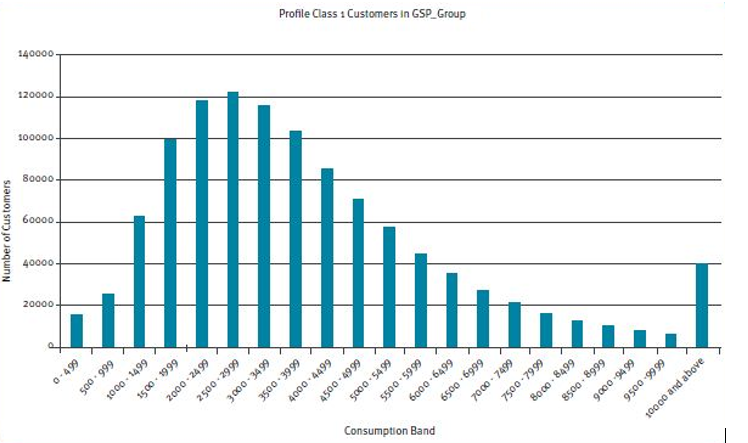Figure 4

## Sample Recruitment

Historically, a small incentive payment has been made to domestic customers who agreed to provide consumption data for the Load Research sample (via a Secondary Meter installation). Following the implementation of Modification P223, Suppliers are now required to provide sample data gathered from customers within their own portfolios. As Secondary meter are removed from the sample, these are replaced by new sites from Supplier portfolios.

## Data Retrieval and Analysis

The Half-Hourly data is collected either remotely or by the PrA (for secondary Metered sample sites) or submitted by Suppliers (or an Agent they have assigned). This data is then loaded into a database and validated; data which does not pass validation is excluded from the analysis, and flagged to the data prover.

This data is then analysed and used to calculate the actual co-efficients that are used to create the load profiles.

A simplified model of the basic data analysis processes are given in Figure 5 below.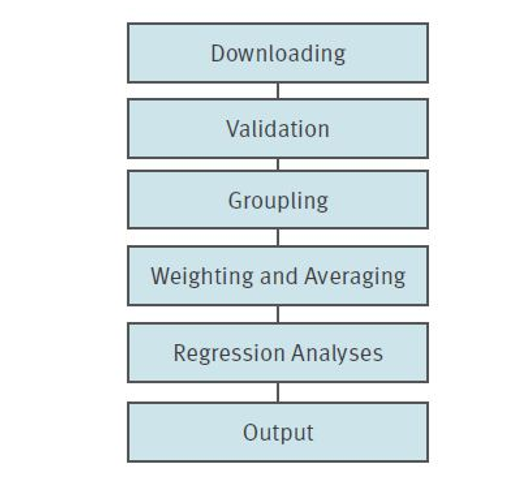Figure 5

## Grouping

After validation, demand data is grouped by Profile Class using the first two digits of the full MPAN. Where an MPAN does not match with the expected Profile Class for the sample site, it will be omitted from the sample.

## Weighting and Averaging

After grouping, it is possible to create a simple average demand for each Half-Hour period of the year for each stratum and GSP Group. These simple averages can be weighted together using the fractions of the population that are in each GSP Group. Figure 6 on the next page shows the weighting and averaging procedure for Profile Class 1, GSP Group _A, Half-Hour 1 on Settlement day 1.

Summating across each GSP Group gives a Group Average Demand (GAD) for that Settlement Period and Settlement Day. Repeating this for each Half-Hour of the year gives a 365 (days) x 48 (half hours) matrix of GADs, which are used in the regression analysis.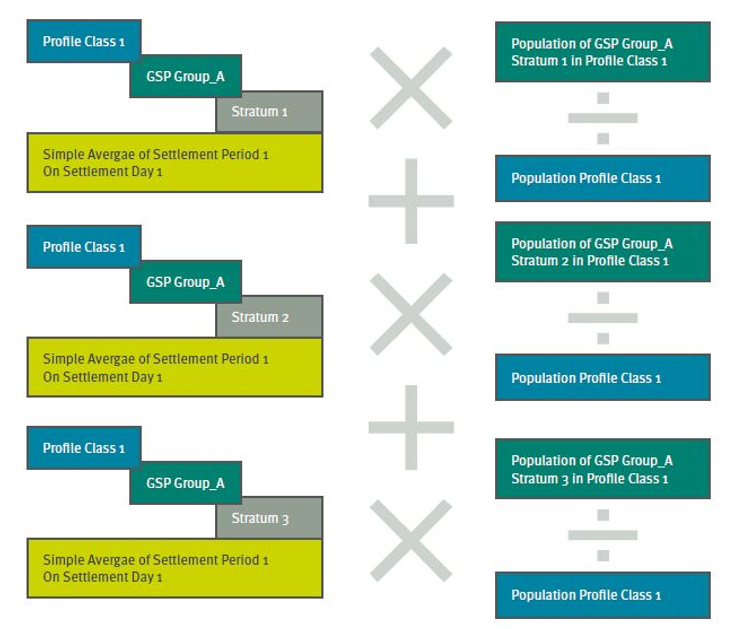Figure 6

## Switched and Base Load for Economy 7 Customers

The P Class 2 GADs are split into switched and base load, using sample customer information on their electric heating and switching regimes (the times when usage is recorded against the meter’s “low” register). Figure 7 depicts a typical switched/base load split.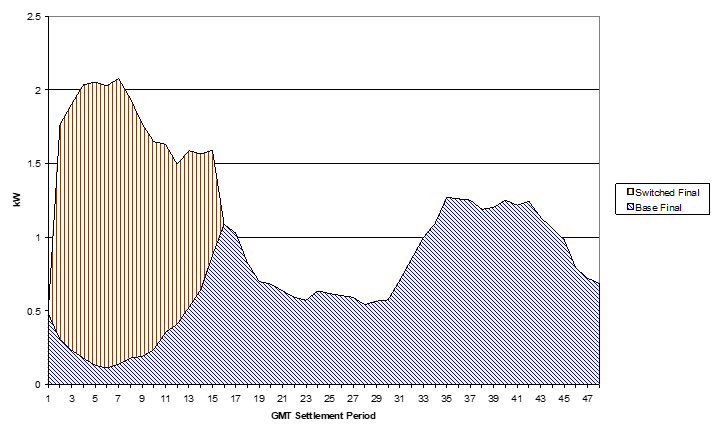Figure 7

## Analyses

### What is linear regression?

The Regression Coefficients used in the Settlement System are multi-linear, meaning they use more than one variable. Up to seven variables, including temperature, sunset and day of the week, can be used. In this example, we will explain regression analysis using a single variable (noon temperature in ºF). This is called simple linear regression, and Figure 8 shows a plot of GADs against noon temperature with a linear trend line (the line of best fit)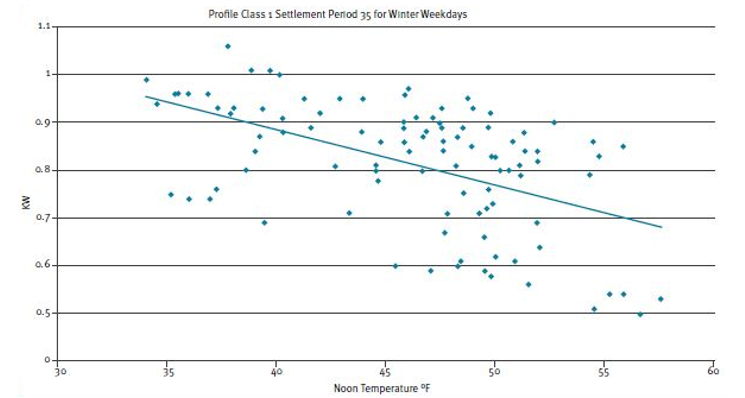Figure 8

Figure 8 shows that for every 1ºF increase in temperature, the trend line falls by a fixed amount. This fixed amount is regarded as a coefficient.

The equation of a straight line can be expressed as:

Y = BX + C

Where Y is the estimate of demand (kW), B is the coefficient, X is the noon temperature and C is a constant (the point where the line intersects with the Y axis when X equals 0). In the above example, the line intersect the Y axis at 1.346 when X equals zero.

Therefore B = -0.0115, and C = 1.346. This means that for every 1ºF increase in temperature, demand falls by around 11.5 W. Using this we can predict the likely demand at any temperature.

So at 50ºF, the estimate of demand will be:

Y = (-0.0115 * 50) + 1.346 = approx. 0.77 kW

The evaluation is demonstrated in Figure 9. The dashed yellow line at 50ºF extends until it meets the regression line; reading this point off on the Y axis gives an estimate of 0.77 kW.

In reality there are multiple variables, so the calculations become more complicated, but the theory is the same.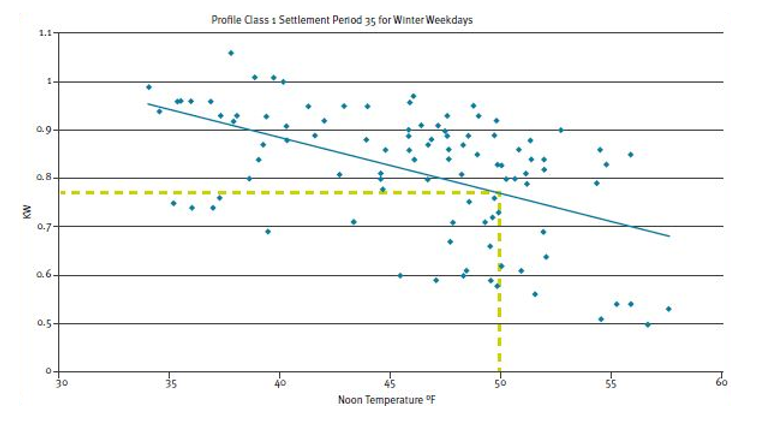Figure 9

### The Variables

This section defines the variables that are used in the regression analysis, and why they are used. There are up to seven variables in each regression, depending on the day type of the regression.

### Noon Effective Temperature (NET)

The above example used noon temperature in ºF. However, in reality the temperature variable used is Noon Effective Temperature (NET). A NET is designed to take account of the residual heat left behind from previous days, and so over a number of days. It is a weighted ºF value of the noon temperature on the day, the previous day, and the day before that. The weights are as follows:

NET = 0.57 * Actual Noon Temperature on Day (ºF) + 0.28 * Actual Noon Temperature on Day-1 (ºF) + 0.15 * Actual Noon Temperature on Day-2 before that (ºF).

Figure 10 shows the England and Wales NET values for a typical year (April to March).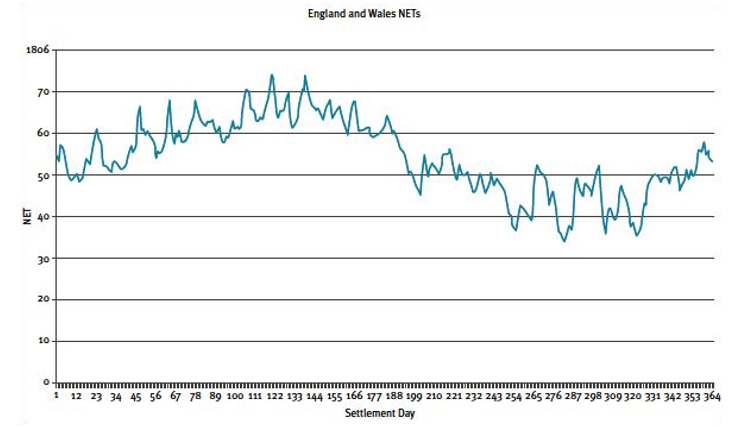Figure 10

The reason for having a temperature variable is obvious: heating load increases when temperatures fall, and decreases when temperatures rise - until any cooling load (e.g. air conditioning) is applied.

### The Sunset Variables

There are two sunset variables used in all the regressions. The sunset variable, which is expressed as minutes before or after 18:00 hours (GMT), is included as this affects illumination (e.g. lights are switched on at sunset).

The other sunset variable is sunset squared. Squaring the sunset variable gives positive values across the year, which can be used to predict seasonal effects. Figure 11 depicts sunset variables for a year (April to March).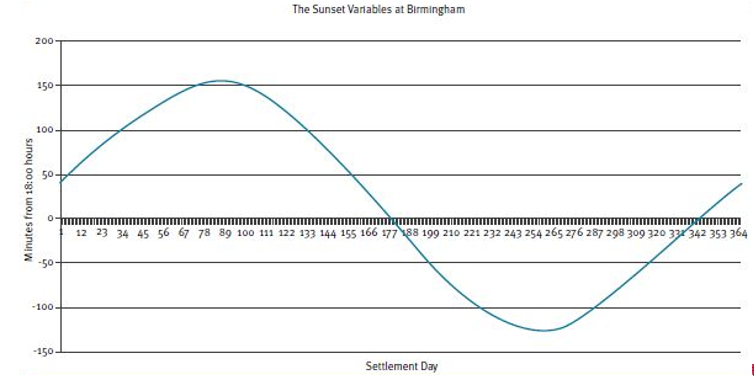Figure 11

### Day of Week Variables

The weekday regressions also include dummy variables. These variables are expressed as 1s and 0s. Four sets of dummy variables are included in the weekday regressions :

Set 1 1 if the day is a Monday else 0

Set 2 1 if the day is a Wednesday else 0

Set 3 1 if the day is a Thursday else 0

Set 4 1 if the day is a Friday else 0

Tuesday is taken to be the standard day and does not get a dummy variable. This means that when evaluating regressions for a Tuesday, the weekday coefficients are ignored.

## Regression Equations and Evaluating Regression Coefficients

Using these variables creates a more complex equation than in the simple linear regression example. The equation for a weekday would be:

Y = (Temperature Coefficient x Temperature Variable) + (Sunset Coefficient x Sunset Variable) + (-Sunset Squared Coefficient x Sunset Squared Variable) + (Monday Coefficient x Monday Variable) + (Wednesday Coefficient x Wednesday Variable) + (Thursday Coefficient x Thursday Variable) + (Friday Coefficient x Friday Variable) + Constant

When evaluating the regression coefficients the relevant variables are used to get an estimate of Y for any Half-Hour.

### The Regression Types

There are fifteen basic regression types which the GAD Matrices are divided into, representing five seasons and three day types. The definitions of the seasons are as follows:

• Winter (Season Id 1): defined as the period from the day of clock change from British Summer Time (BST) to Greenwich Mean Time (GMT) in October, up to and including the day preceding the clock change from GMT to BST in March;

• Spring (Season Id 2): defined as the period from the day of clock change from GMT to BST in March, up to and including the Friday preceding the start of the Summer period;

• Summer (Season Id 3): defined as the ten-week period, preceding High Summer, starting on the sixteenth Saturday before the August Bank Holiday;

• High Summer (Season Id 4): defined as the period of six weeks and two days from the sixth Saturday before August Bank Holiday up to and including the Sunday following the August Bank Holiday; and

Each season is divided into Week Days, Saturdays and Sundays. Figure 12 on the next page shows the relationship between the GADS and the Regression Coefficients :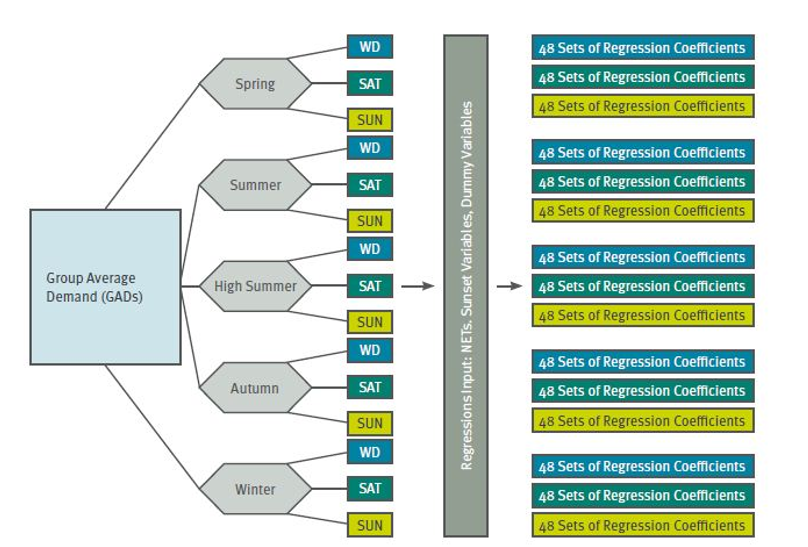Figure 12

### The Regressions and “Special Days”

The regressions are performed on a Half-Hourly basis within each of the fifteen basic day-types, using the relevant GADs and variables. However, some days, such as Bank Holidays, are unlike other days. In order to create Regression Coefficients for these days, the Sunday Coefficients for the relevant season are used and an adjustment is made to the constant term using information from the GADs for the relevant day. The regression output is Regression Coefficients for each Profile Class, Season, Day-type, Half-Hour, Coefficients and the Constant. An example is given in Table 1 below: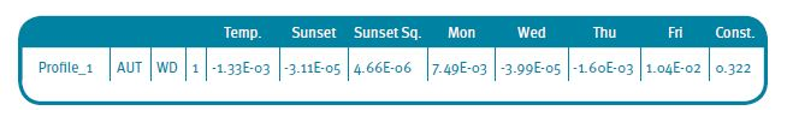Table 1

### Evaluating the Coefficients

Evaluation of the coefficients will usually occur at out-turn NETs and Sunset Variables for the day that is being estimated. Figure 13 shows how the coefficients evaluate to form a profile.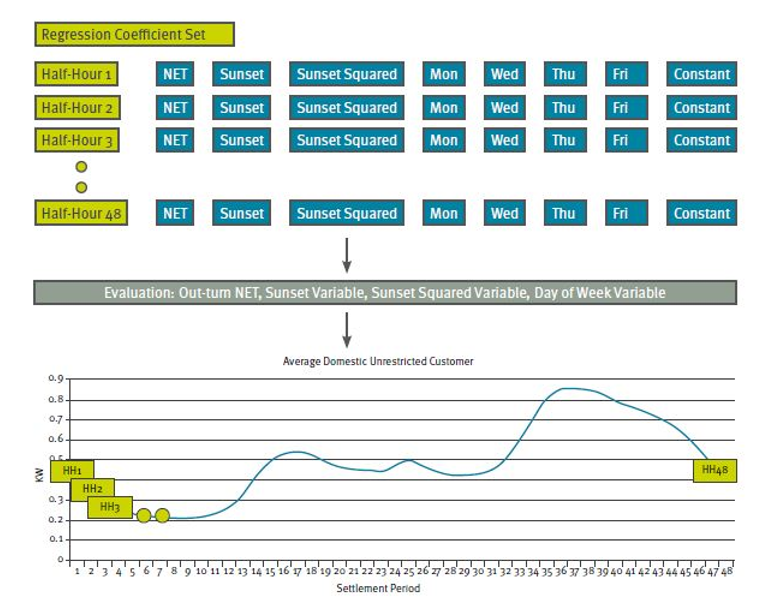Figure 13

An example evaluation, using the above coefficients, for a Wednesday, with a NET of 52ºF and a Sunset Variable of -13 is given in Table 2 below: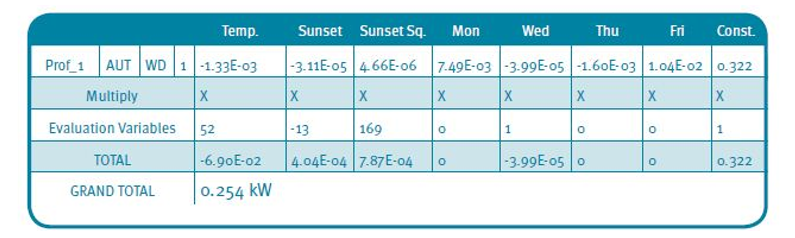Table 2

### Algorithmic Profiling

The Regression Coefficients for Economy 7 switched load profiles undergo another step called algorithmic profiling. This process allows the coefficients to model switching regimes that are less than or greater than seven hours, or even switching regimes that are split across the day.

Figure 14 shows example evaluated Regression Coefficients for regimes of various lengths. The procedure ensures that the area under each curve remains the same.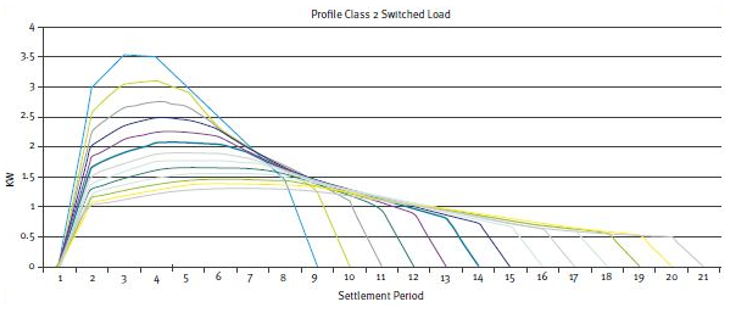Figure 14

## Additional data provided by the PrA

### Group Average Annual Consumption (GAAC)

Using ten-year average NETs and sunset variables for each GSP Group, the relevant Regression Coefficients are evaluated for every day in a Settlement, for each Profile Class and GSP Group. Summing the evaluations (within each Profile Class and region) and dividing by 2 creates a Group Average Annual Consumption (GAAC) in kWh. The GAACs are used for calculation of Profile Coefficients in the Settlement System and are provided in MWh. An example of a GAAC is given below:

 "GSP" "Profile_Class" "Type" "GAAC" "C" 1 "TOTAL" 3.740 MWh

### Profile Coefficients

A Profile Coefficient is an estimate of the fraction of yearly consumption within each Settlement period. It can refer to a fraction of the whole year, or the fraction of consumption in a Settlement period relating to a single Meter register to the yearly consumption for that register. Profile Coefficients are calculated as follows:

Evaluated Regression Equation / (GAAC * 2000)

The GAAC is multiplied by 2000 to convert it from MWh to kW, so that:

kW per Half-Hour/ kW per year

The use of Regression and Profile Coefficients are discussed in the next section, which describes how load profiles are used to estimate Half-Hourly consumption from a Meter advance.

# SECTION C

## Using Load profile data to Estimate Half-Hourly Consumption from a meter advance

The Settlement system uses load profiles to estimate Half-Hourly consumption for all supply market customers, with numerous metering configurations on a daily basis for each supplier. Operation of the NHH Settlement system is undertaken by the BSC and Party Agents.

To make this simpler, let’s consider how the load profiles can be used to estimate the Half-Hourly usage for a single supply market customer, with a single meter register.

Example 1: A domestic unrestricted customer in London with a quarterly meter advance of 1,000kWh (for period 1 April – 30 June 2021)

Step 1: Evaluate the Regression Coefficients using the NETs for the relevant period, and include London Sunset Variables and other variables as appropriate. This will calculate the Estimated Regional Average Demands per Customer (ERADPCs).

Figure 15 shows the evaluated Regression Coefficients for Profile Class 1: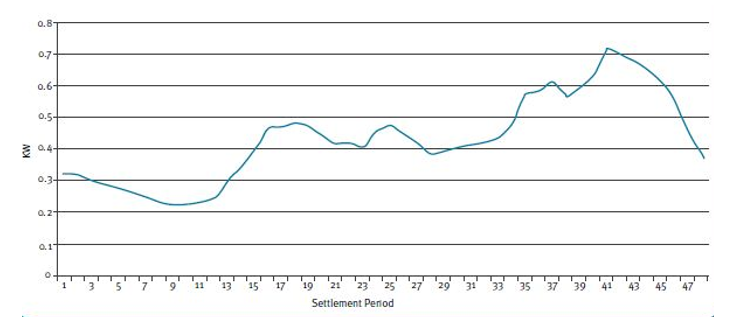Figure 15

Step 2: Calculate Profile Coefficients for the Meter advance period, using the GAAC (see Figure 16):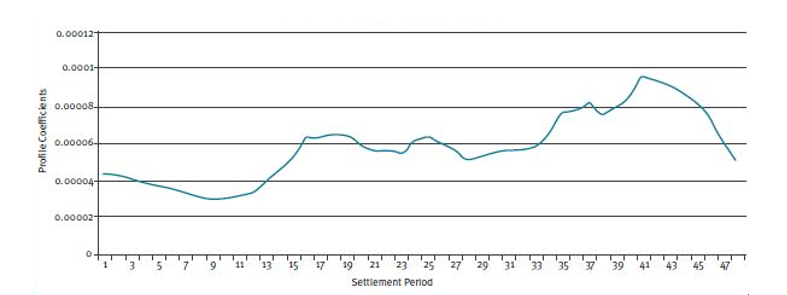Figure 16

Step 3: Calculate an Annualised Advance (AA)

To calculate an Annualised Advance you simply divide the Meter advance by the sum of the Profile Coefficients over the period of the advance (1 April -30 June 2021) in this case:

= 4522 kWh (Estimation of customer’s annual consumption)

Step 4: Allocate the Calculated volume to each day

To allocate the calculated volume to each day, simply multiply the Profile Coefficients by the AA. Figure 17 shows the allocation of the AA to the Profile Coefficients for the 1 April.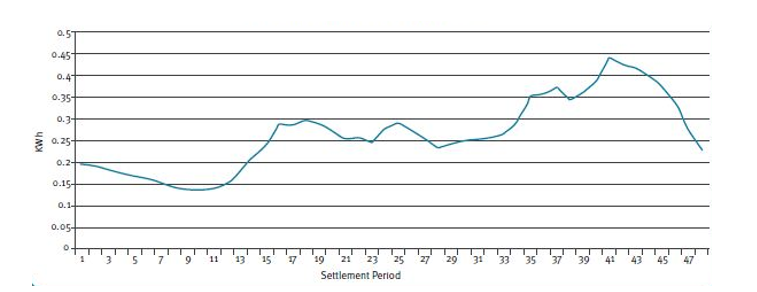Figure 17

The customers Meter advance has now been split into Half-Hourly consumption without the need of Half-Hourly metering. The daily consumption estimate over the Meter reading period can be seen in Figure 18.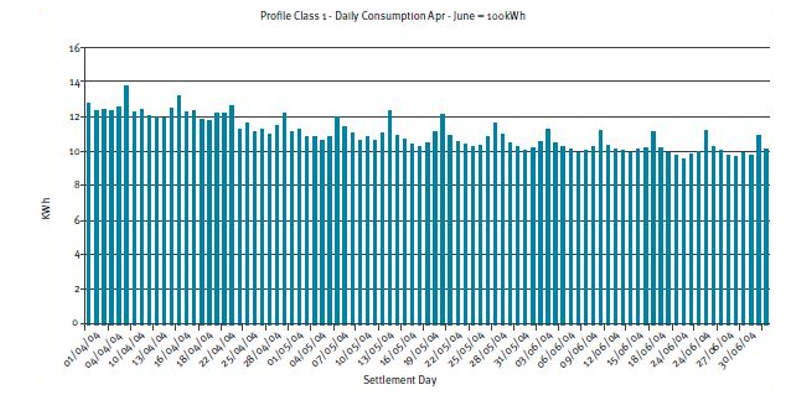Figure 18

In practice, the true profile for an individual customer would differ to the profile shape, as this profile shape is of an “average customer” and all customers’ usage patterns differ. In reality, all domestic unrestricted customers in a GSP region have AAs calculated and aggregated before the volume is allocated.

If there is no Meter advance for a customer, an Estimated Annual Consumption (EAC) is – calculated using historical Meter Reading information. This is used in Settlements until the customers meter has been read. At this level, the profile should give a reasonable estimate of a Supplier’s take for each Half-Hour.

In reality, many customers have metering configurations with more than one register. The configurations are referred to as Standard Settlement Configurations (SSC’s) and the registers as Time Period Registers (TPRs). For each SSC, the approximate fraction of consumption on each register is calculated; this is referred to as the Annual Fraction of Yearly Consumption (AFYC).

Example 2: A domestic Economy 7 customer in London on an 00:30–07:30 GMT switching regime (01:30-08:30 BST), who has a low register advance of 500 units and a normal register advance of 1000 units, for the period 1 April – 30 June 2021 (note this example also demonstrate how switched and base load coefficients are used).

Step 1: Evaluate the Regression Coefficients for switched and base load using the NETs for the relevant period, and London Sunset Variables. Figure 19 shows using a stacked bar chart of the evaluated Regression Coefficients for Profile Class 2 for the 1 April 2004.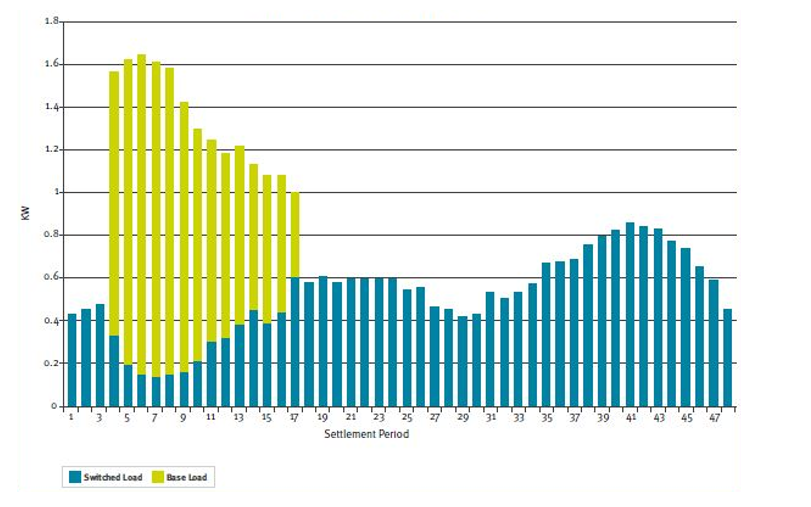Figure 19

Step 2: The Basic Period Profile Coefficients (BPPC) for switched and base load are calculated in the GAAC. However, the two sets of Profile Coefficients need to be combined in the correct proportions. Figure 20 shows Combined Period Profile Coefficients (CPPCs) for 1 April.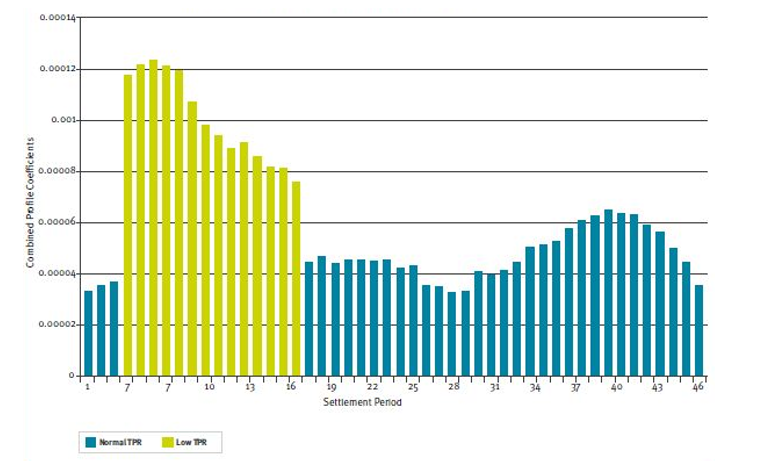Figure 20

Step 3: Next, divide the coefficients on each register by the corresponding AFYC for that register (0.4 low and 0.6 normal are used for this example). This is referred to as ’chunking’ and creates Period Profile Class Coefficients (PPCCs). Figure 21 shows the ‘chunked’ coefficients for 1 April: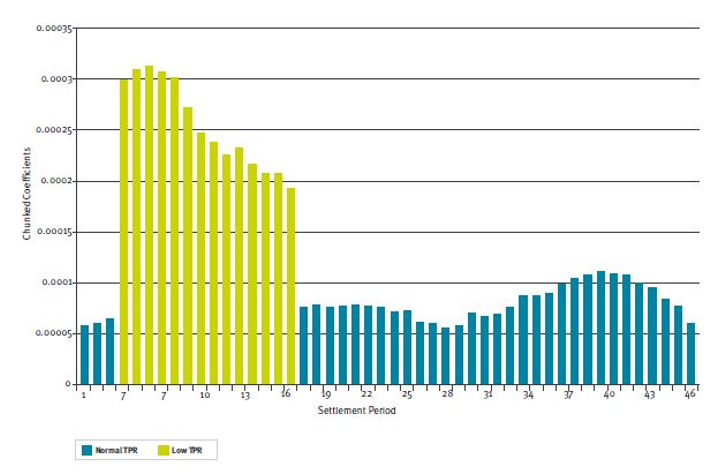Figure 21

Step 4: Calculate AA for each register by dividing the annual advances by the sum of the ‘chunked’ coefficients for the register over the reading period. In this example:

Low AA = 500/0.174 = 2869

Normal AA = 1000/0.232 = 4304

Step 5: Multiply the ‘chunked’ coefficients (PPCC’s) by the relevant AA to give Half-Hourly consumption estimates for each register. Figure 22 shows the volumes allocated to each TPR for 1 April.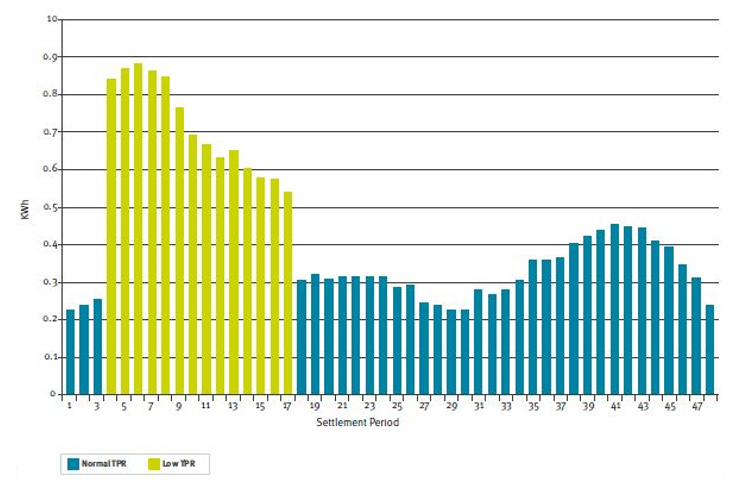Figure 22

As before, these calculations would in reality use aggregated AAs and EACs for all customers on the same SSC in each GSP Group for each Supplier.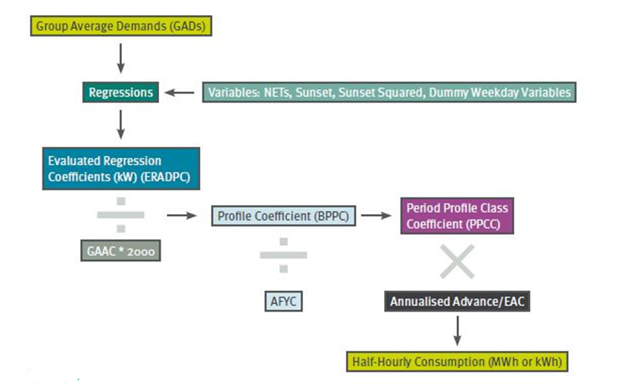Figure 23 summarises the calculations. The SSCs, TPRs, switching times, AFYCs, and other information are contained in the Market Domain Data (MDD):

Figure 23

## The BSC Agents

All the calculations above are carried out by BSC Agents and BSC Party Agents. The following is a brief overview of the agents, and their roles in NHH Settlement as they relate to load profiles.

The PrA calculates the Regression Coefficients, GAACs and ‘default’ Profile Coefficients (coefficients to be used when a true coefficient cannot be calculated).

### Non Half-Hourly Data Collectors (NHHDCs)

The NHHDCs read the NHH Meters and calculate AAs and EACs using the Daily Profile Coefficients (DPCs) (sum of PPCCs for the Settlement day and Settlement period) produced by the Supplier Volume Allocation Agent (SVAA). They provide the AAs together EACs to the Non Half-Hourly Data Aggregators (NHHDAs).

### Non Half-Hourly Data Aggregators (NHHDAs)

The NHHDAs aggregate the AAs and EACs by Supplier, GSP Group, Profile Class (PC), Line Loss Factor (LLF), Standard Settlement Configuration (SSC), and Time Pattern Regime (TPR) to create a Supplier Purchase Matrix (SPM), which is used by the SVAA.

### The Supplier Volume Allocation Agent (SVAA)

The SVAA uses the Regression Coefficients to calculate DPCs for each SSC and TPR combination in the MDD. The SVAA allocates the aggregated volumes given in the SPM to the applicable Settlement period using the Period Profile Class Coefficients (PPCCs). The SVAA also calculates the AFYCs and provides the MDD.

### Supplier Metering Registration Agents (SMRAs)

The SMRA provides information to the other agents on Metering Systems and their Suppliers within each GSP Group.

Figure 24 shows in a simplified format the relationships between the Non Half-Hourly Agents.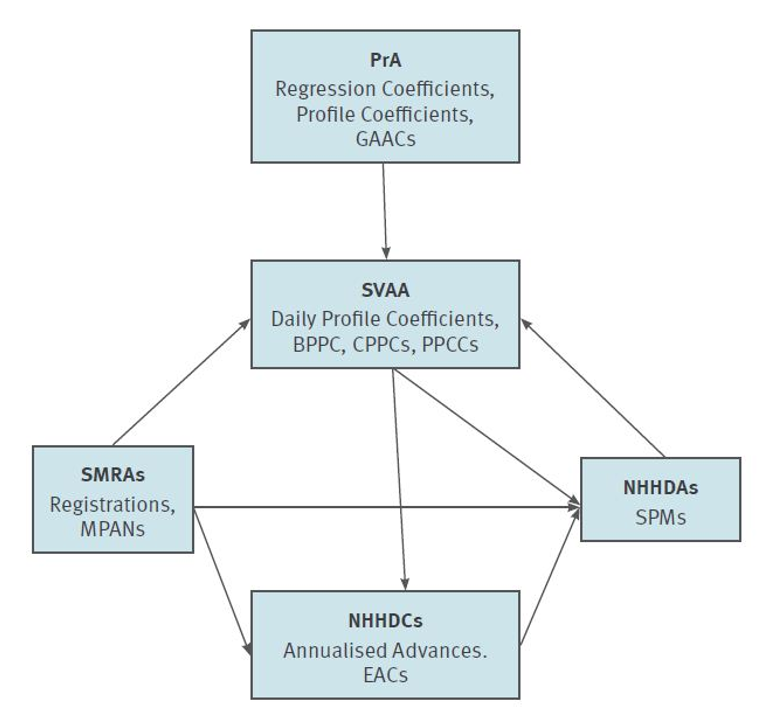Figure 24

## Settlement Runs

The daily Settlement runs calculate the Profile Coefficients for the day. The Profile Coefficients for previous days are updated with AAs calculated using the latest Meter reading information.

After a 14 month period, Final Reconciliation (RF) for the settlement day occurs when hopefully most, if not all, supply customers’ meters have been read at least once.

## Profiling Error

Sources of load profiling error occur at every stage; however, these errors fall into two main categories:

### Profile Estimation Errors

The two main sources of Profile Estimation Error are:

Sampling Error: Sampling Errors are inherent in all samples, but tends to reduce as the sample size increases.

Regression Error: This is unavoidable, as shown in Figures 8 and 9 above. The vertical distance between the trend line and the data points can be described as the regression error. These are also sometimes referred to as residual demands.

### Profiling Process Errors

The following are a few of the Profiling Process Errors:

• Application of national profiles to GSP Groups

• Incorrect assignment of customers to profile

• Incorrect AAs and EACs

## GSP Group Correction Factors

When all Suppliers’ profiled Half-Hourly consumptions are summated across a GSP Group, the total can be compared to the actual metered take for the Group (after taking account of Half-Hourly metered data).

Dividing the profiled take into the actual take creates a GSP Group Correction Factor (GSPGCF). This factor is applied to each Supplier’s individual profiled take in order to correct the profiled error.

## Market-wide Half Hourly Settlement (MHHS)

Following the publication in 2021 of Ofgem’s Full Business Case for their Significant Code Review on Settlement Reform, it was agreed to introduce half-hourly settlement (HHS) on a market-wide basis. Once this is live, load profiles for non Half-Hourly settlement will not be required as non Half-Hourly settlement will cease to exist.

Current expected timeline for MHHS are shown in Figure 25 below:Figure 25

As part of the movement to MHHS, a new Load Shaping Service (LSS) will be introduced. This will be responsible for calculating energy consumption (import and export) Load Shapes for a number of defined Categories of Metering Systems.

The LSS will use validated actual Settlement Period (SP) level data accessed from the Processing Service (Smart) (PSS). The Load Shapes will then be used to convert Register Readings (RRs) or Daily Consumption values into Settlement Period level data. In addition, the Load Shape data will be used to estimate invalid SP level data for smart Meters, and default where data is missing or unavailable.